Function Repository Resource:

# ApproximateVertexCover

Find a vertex cover not much larger than the minimal cover

Contributed by: Wolfram Staff (original content by Sriram V. Pemmaraju, Steven S. Skiena and Daniel Lichtblau)
 ResourceFunction["ApproximateVertexCover"][g] returns a vertex cover of graph g constructed using the greedy algorithm. ResourceFunction["ApproximateVertexCover"][g,vlist] tries to improve the vertex cover vlist.

## Details and Options

Possible forms for vlist are:
 All all vertices of g Automatic endpoints of the largest independent edge set of g {v1,v2,…} specified vertices vi
The default value of vlist is All.
The greedy heuristic deletes edges that are incident on the maximum degree vertex in vlist and repeats the procedure until all edges of g are covered.
The greedy algorithm is a natural heuristic for constructing a vertex cover, but it can produce poor vertex covers.
ResourceFunction["ApproximateVertexCover"][g,Automatic] uses a vertex cover constructed with and is guaranteed to be within two times the minimal.
ResourceFunction["ApproximateVertexCover"][g,vlist] does not necesserily improve the size of the vertex cover.
ResourceFunction["ApproximateVertexCover"] works with undirected graphs, directed graphs, multigraphs and mixed graphs.
ResourceFunction["ApproximateVertexCover"] works with large graphs.
ResourceFunction["ApproximateVertexCover"] takes the Method option, with possible values as follows:
 Automatic pick the method automatically "Greedy" greedy heuristic with dynamic updates "IndexedHeap" greedy heuristic with tracking vertices in an indexed heap "GreedyStatic" simplified greedy heuristic
With (default), ResourceFunction["ApproximateVertexCover"] switches from the "Greedy" method to "IndexedHeap" for larger graphs.
The simplified greedy heuristic "GreedyStatic" is generally faster than other methods, but can give somewhat larger covers.

## Examples

### Basic Examples (2)

Find an approximate vertex cover:

 In:=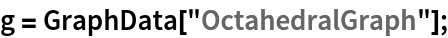In:=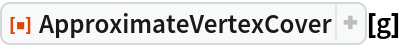Out=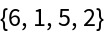Show the cover:

 In:=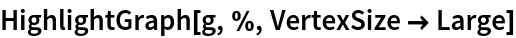Out=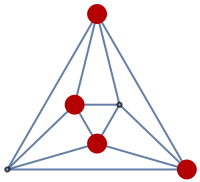### Scope (5)

Use the greedy heuristic to find an approximate vertex cover starting from the full vertex set:

 In:=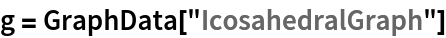Out=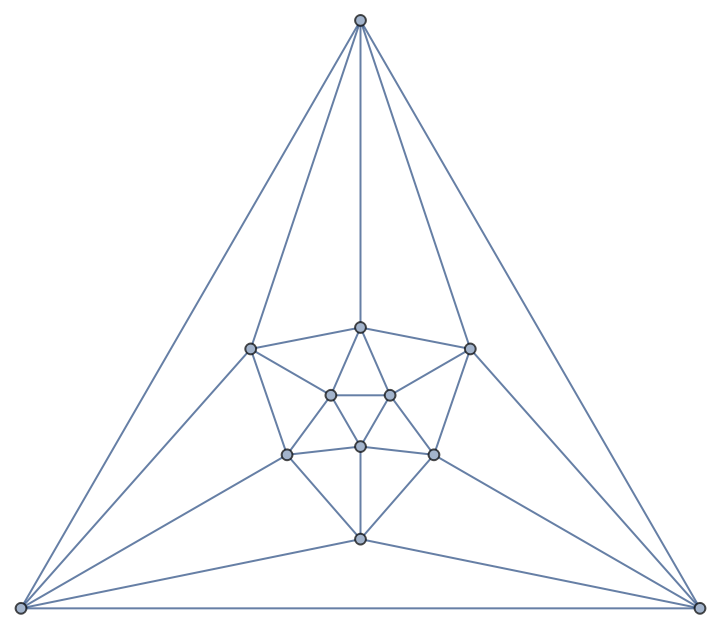In:=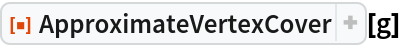Out=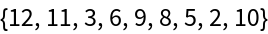This is the same as:

 In:=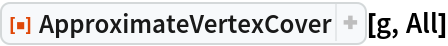Out=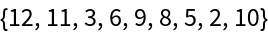Improve a vertex cover:

 In:=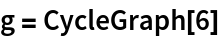Out=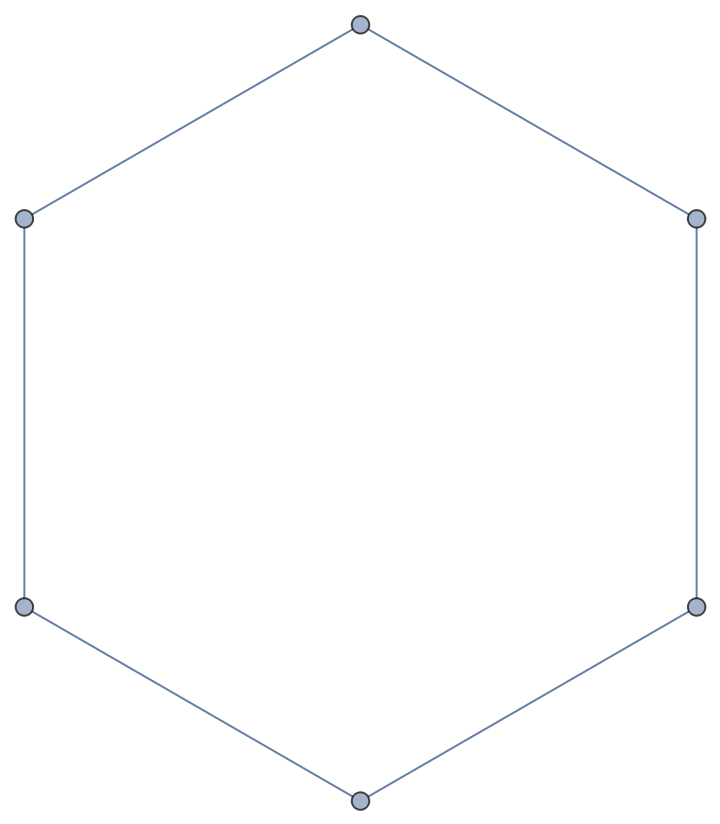In:=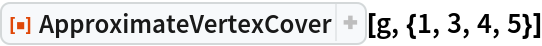Out=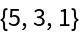Use endpoints of the largest independent edge set of g as a starting point:

 In:=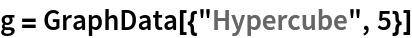Out=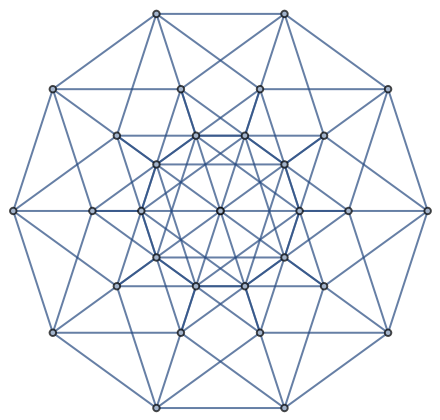In:=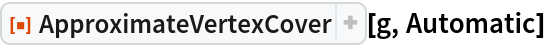Out=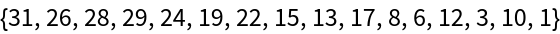ApproximateVertexCover works with undirected graphs:

 In:=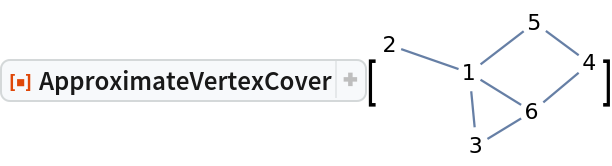Out=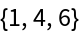Directed graphs:

 In:=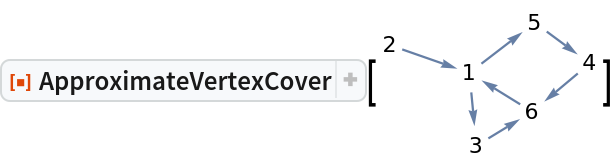Out=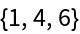Multigraphs:

 In:=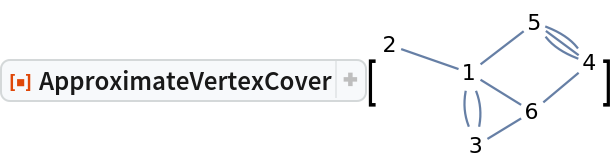Out=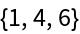Mixed graphs:

 In:=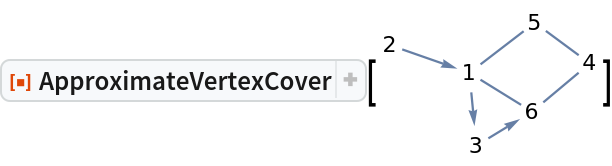Out=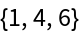ApproximateVertexCover works with large graphs:

 In:=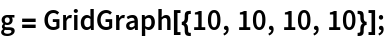In:=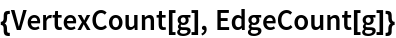Out=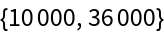In:=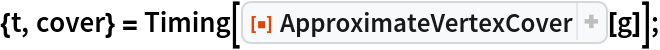In:=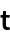Out=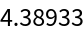In:=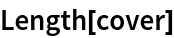Out=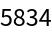In:=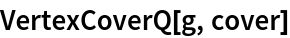Out=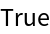### Options (2)

#### Method (2)

The dynamic greedy heuristic is the default for relatively small graphs:

 In:=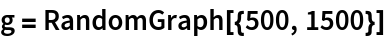Out=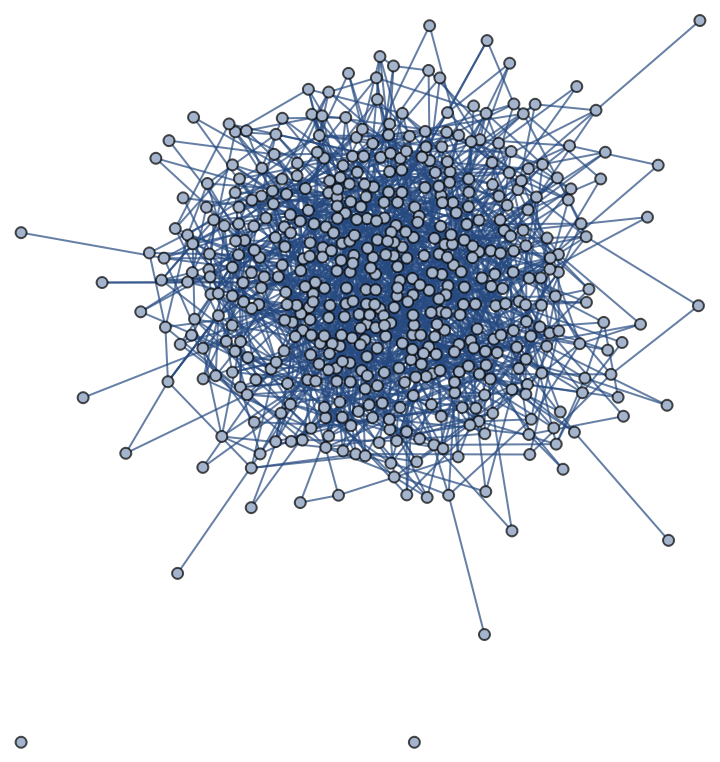In:=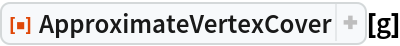Out=In:=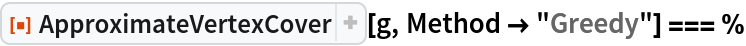Out=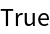Method"IndexedHeap" is most advantageous for larger graphs, for which it is the default:

 In:=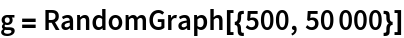Out=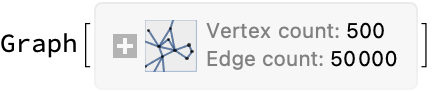In:=Out=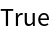For relatively smaller graphs, Method"Greedy" can be faster, while giving a comparable cover:

 In:=Out=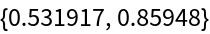In:=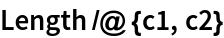Out=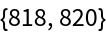In:=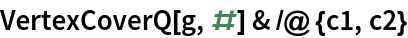Out=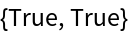Method"GreedyStatic" is faster for both small and large graphs, at the expense of giving somewhat larger covers:

 In:=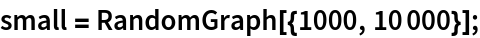In:=In:=In:=In:=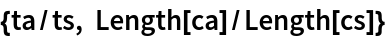Out=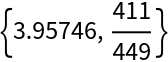In:=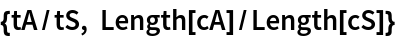Out=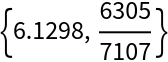### Properties and Relations (7)

The result of ApproximateVertexCover can be tested by VertexCoverQ:

 In:=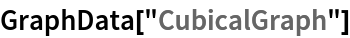Out=In:=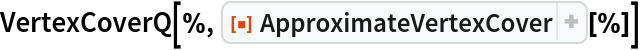Out=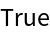ApproximateVertexCover may return results similar to FindVertexCover:

 In:=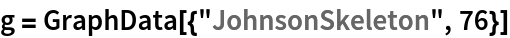Out=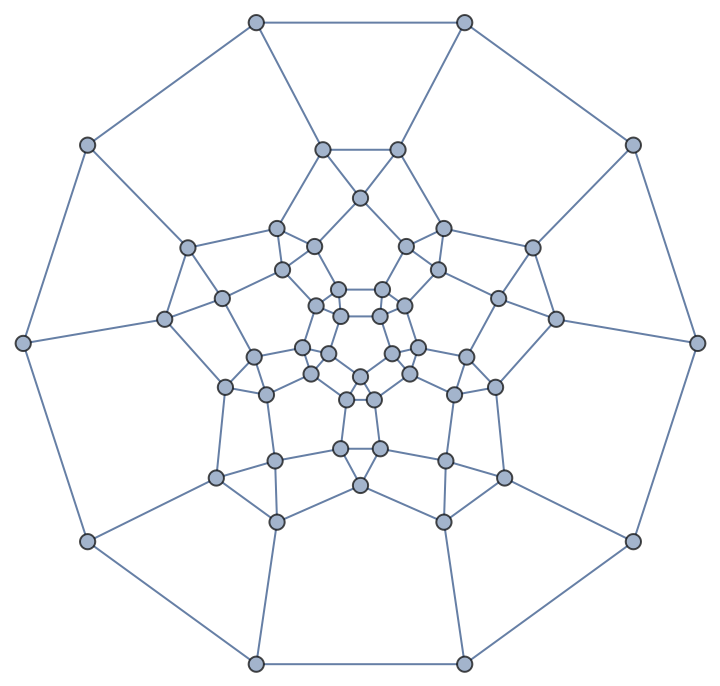In:=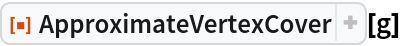Out=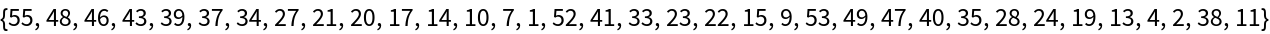In:=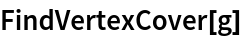Out=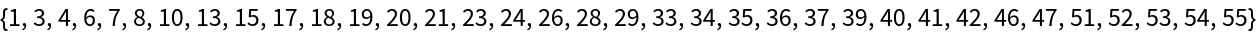In:=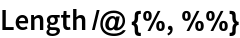Out=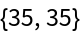However, it does this in less time:

 In:=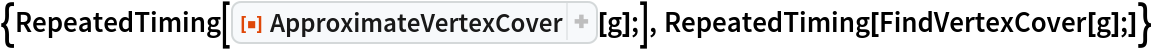Out=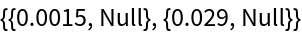However, ApproximateVertexCover may also return covers of a larger size compared to FindVertexCover:

 In:=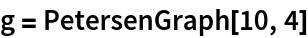Out=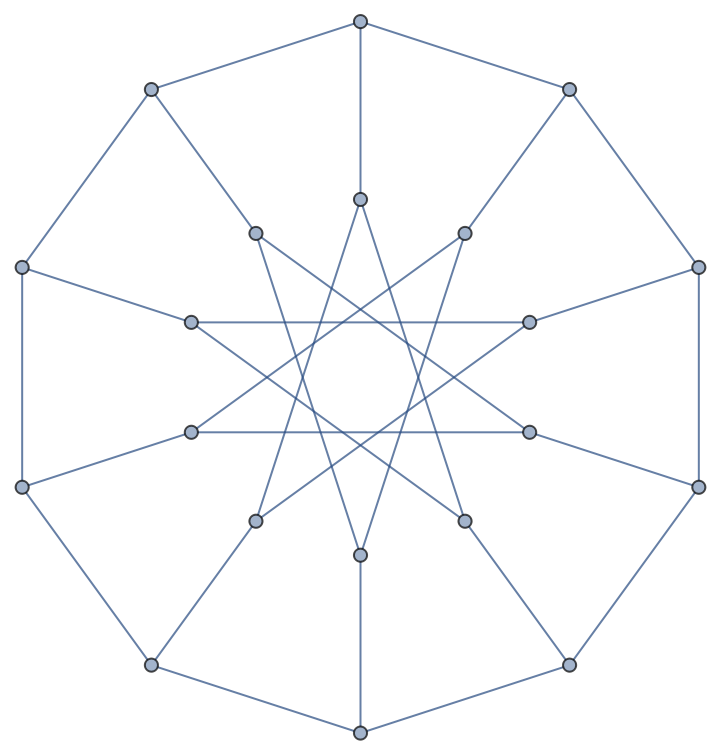In:=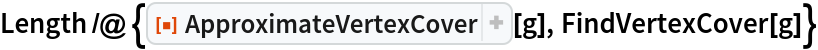Out=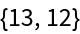Using the Automatic value for vlist may give a smaller cover:

 In:=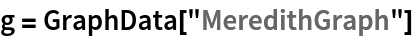Out=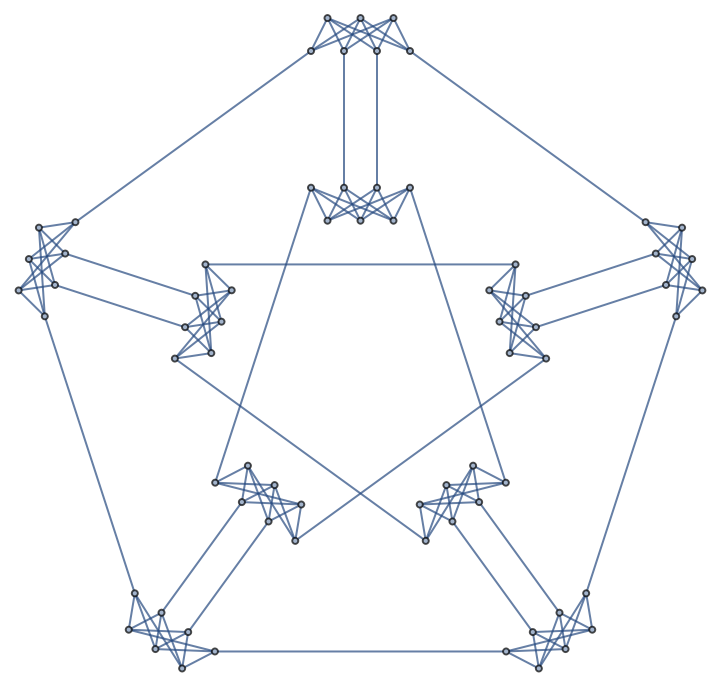In:=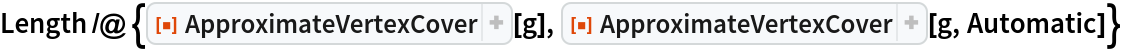Out=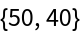ApproximateVertexCover[g,All] may return covers that are equivalent, smaller or larger than ones given by ApproximateVertexCover[g,Automatic]:

 In:=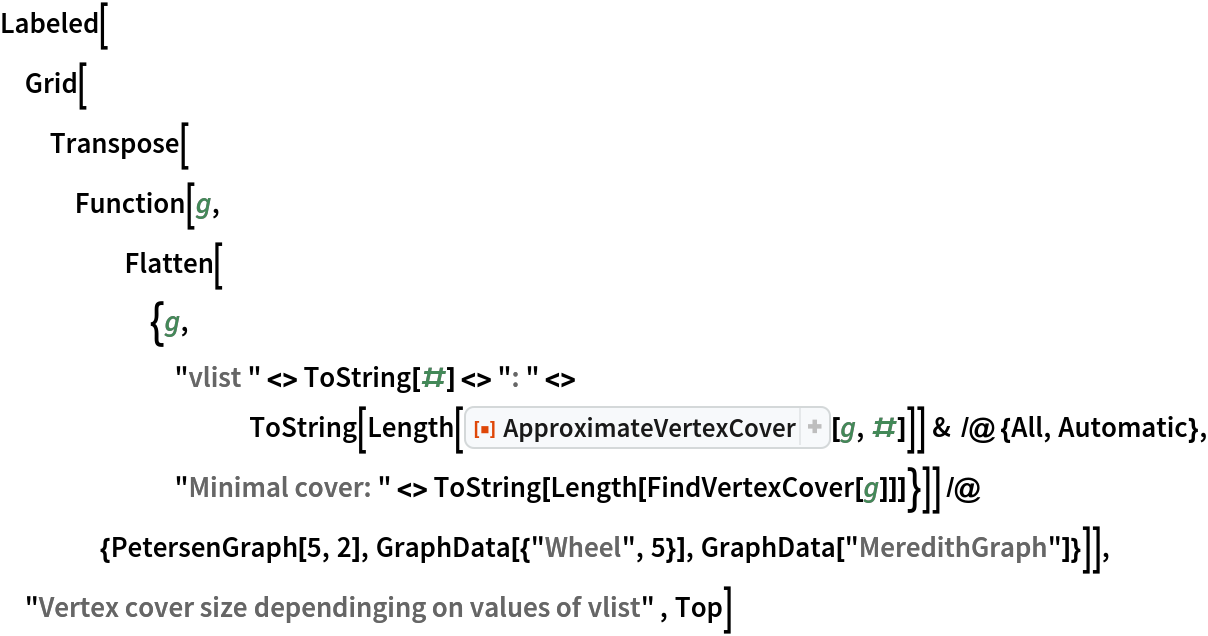Out=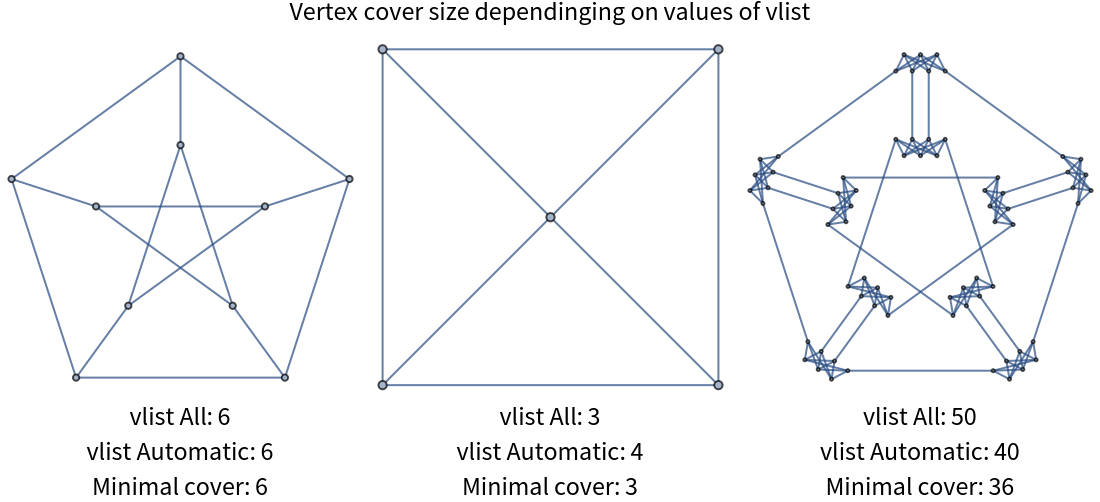Although the algorithm in ApproximateVertexCover[g,Automatic] is guaranteed to give results not exceeding the minimal size by a factor of two, in practice the difference is often smaller:

 In:=Out=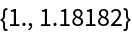Similarly, although ApproximateVertexCover[g] comes with no performance guarantees, in practice it often gives results that are not much worse than the minimal:

 In:=Out=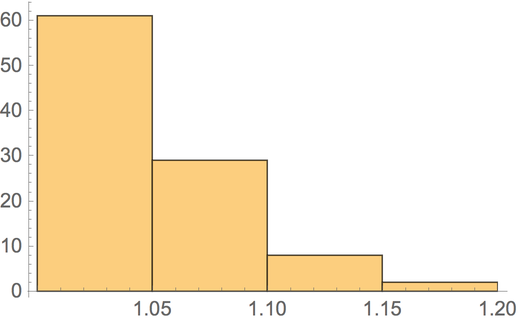### Possible Issues (2)

ApproximateVertexCover[g,{v1,v2,}] works only if {v1,v2,} is a vertex cover:

 In:=In:=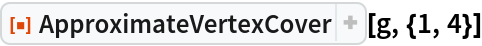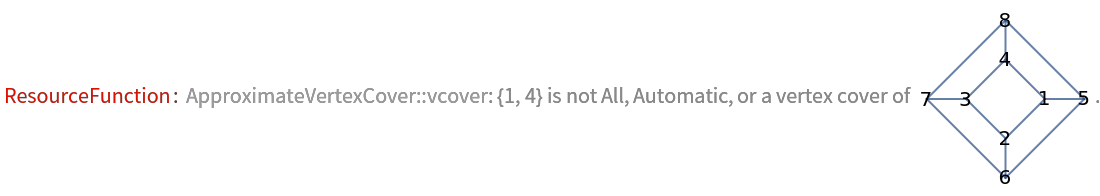Out=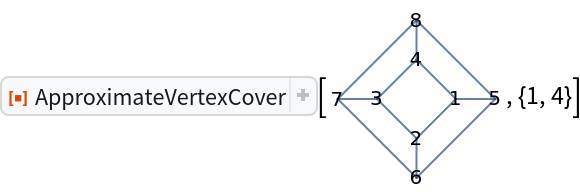If you do not have a suitable vertex cover to improve, try using ApproximateVertexCover[g] without vlist:

 In:=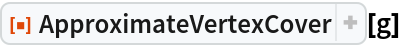Out=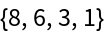## Version History

• 2.0.0 – 02 October 2020
• 1.0.0 – 21 September 2020